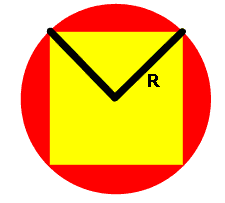From: Lisa A square is in scribed in a circle. Determine the percent of the  circle's area that is outside the square. That is, determine the  shaded area as a percent of the circle's total area. Hi Lisa. I've drawn a diagram of a square (yellow) inscribed in a circle (red) and I've also drawn two radii (length R) in black that will be helpful in understanding this problem.Let's allow C = area of circle and S = area of square. Then what you are seeking is the percentage P = 100(C-S)/C. I'm sure you know the formula for the area of a circle if its radius is R, but can you figure out the area of the square if you are given the length from a corner to the center of the square? Here's a hint: because of symmetry, the rwo radii I've drawn are at right angles. That means you have four equal triangles all of whose base length and height you know! Hope this helps! Stephen La Rocque>